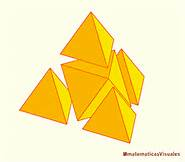# Constructing a tetrahedron

Probability Level 3

For this problem, we will define a "unit polyhedron" to be a polyhedron of edge length one.The picture to the right shows how a larger tetrahedron can be built from four unit tetrahedra and one unit octahedron.

Amanda wishes to construct a much larger tetrahedron with many more unit tetrahedra and octahedra. In the end, she builds one using exactly 364 unit octahedra and some unit tetrahedra.

How many unit tetrahedra did she use?

Assumption: The final construction is one solid tetrahedron, with nothing extra sticking out, and nothing missing (no holes). No unit octahedra or tetrahedra are cut in any way.

Image credit: http://www.matematicasvisuales.com/

×